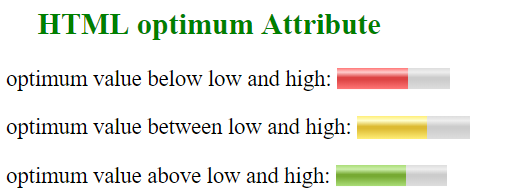# HTML | optimum Attribute

• Difficulty Level : Basic
• Last Updated : 03 Jul, 2021

The optimum attribute in HTML indicates the optimal numeric value for the gauge. It must be within the range i.e between min and max. When it is used with the low and high attribute, it gives an indication where along the range is considered preferable.
Usage: It is used with the <meter> element.
Syntax:

`<meter optimum = "value"></meter>`

Attribute Values:  It contains the floating point number which represent the optimal value of the gauge.

Attention reader! Don’t stop learning now. Get hold of all the important HTML concepts with the Web Design for Beginners | HTML  course.

Example:

## html

 ```<``html``>``    ``<``head``>``        ``<``title``>HTML optimum Attribute``    ````    ``<``body``>``        ` `        ``<``h2` `style` `= ``"color: green;"``>``            ``HTML optimum Attribute``        ````        ` `        ` `<``p``>``            ``optimum value below low and high:``            ``<``meter` `value``=``"0.6"` `max``=``"0.9"` `min``=``"0.1"``                   ``optimum``=``"0.1"` `high` `= ``"0.5"` `low` `= ``"0.2"``>``            ````        ``` `        ` `        ` `<``p``>``            ``optimum value between low and high:``            ``<``meter` `value``=``"0.6"` `max``=``"0.9"` `min``=``"0.1"``                   ``optimum``=``"0.4"` `high` `= ``"0.5"` `low` `= ``"0.2"``>``            ````        ``` `        ` `        ` `<``p``>``            ``optimum value above low and high:``            ``<``meter` `value``=``"0.6"` `max``=``"0.9"` `min``=``"0.1"``                   ``optimum``=``"0.6"` `high` `= ``"0.5"` `low` `= ``"0.2"``>``            ````        ``` `    `````Supported Browsers: The browser supported by optimum attribute are listed below: